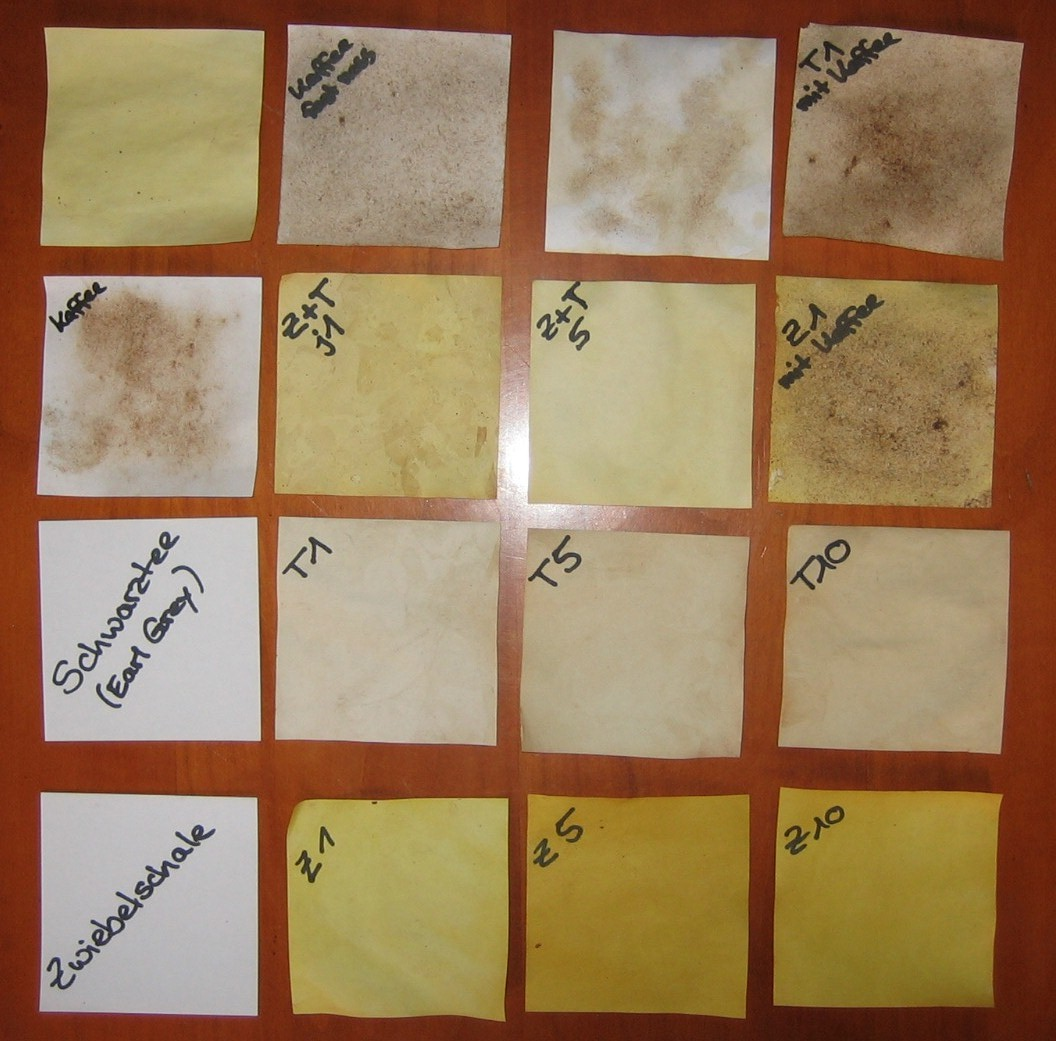Edit Diskussion History

LarpPapier/Diskussion

Ein Beispiel:• Page.execute = 0.158s
• _xapianProcess = 0.000s
• _xapianQuery = 0.139s
• _xapianSearch = 0.140s
• getACL = 0.002s
• getPageCount = 0.008s
• init = 0.001s
• load_multi_cfg = 0.000s
• run = 0.173s
• send_page = 0.172s
• send_page_content = 0.158s
• send_page|1 = 0.158s
• total = 0.174s